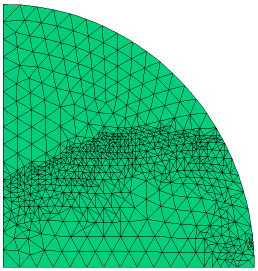﻿ Sample Problems > Usage > Mesh_Control > front

# front

Navigation:  Sample Problems > Usage > Mesh_Control >

# front{ FRONT.PDE

This example demonstrates the use of the FRONT statement

to create a dense mesh at a moving front.

The FRONT command is used to force mesh refinement wherever the

concentration variable passes through a value of 0.5.

The problem is the same as CHEMBURN.PDE.

}

 title 'FRONT statement in Chemical Reactor'   select   painted     { make color-filled contour plots }   variables  Temp (threshold=1)  C (threshold=1)   definitions  Lz = 1  r1=1  heat=0  gamma = 16  beta = 0.2  betap = 0.3  BI = 1  T0 = 1  TW = 0.92 { the very nasty reaction rate: }  RC = (1-C)*exp(gamma-gamma/Temp)    xev=0.96   { some plot points }  yev=0.25   initial value  Temp=T0  C=0equations

Temp:  div(grad(Temp)) + heat + betap*RC = dt(Temp)

C:  div(grad(C)) + beta*RC = dt(C)

boundaries

region 1

start (0,0)

{ a mirror plane on X-axis }

natural(Temp) = 0

natural(C) = 0

line to (r1,0)

{ "Strip Heater" at fixed temperature }

{ ramp the boundary temp in time, because  discontinuity is costly to diffuse }

value(Temp)=T0 + 0.2*uramp(t,t-0.05)

natural(C)=0               { no mass flow on strip heater }

arc(center=0,0) angle 5

{ convective cooling and no mass flow on outer arc }

natural(Temp)=BI*(TW-Temp)

natural(C)=0

arc(center=0,0) angle 85

{ a mirror plane on Y-axis }

natural(Temp) = 0

natural(C) = 0

line to (0,0) to close

time 0 to 1

{ FORCE CELLS TO SPAN NO MORE THAN 0.1 ACROSS C=0.5 }

front(C-0.5, 0.1)

plots

for cycle=10                 { watch the fast events by cycle }

grid(x,y)

contour(Temp)

contour(C) as "Completion"

for t= 0.2 by 0.05 to 0.3       { show some surfaces during burn }

surface(Temp)

surface(C) as "Completion"

histories

history(Temp,C) at (0,0) (xev/2,yev/2) (xev,yev) (yev/2,xev/2) (yev,xev)

end